Nonequivalent Groups Analysis

# Nonequivalent Groups Analysis

## Analysis Requirements

The design notation for the Non-Equivalent Groups Design (NEGD) shows that we have two groups, a program and comparison group, and that each is measured pre and post. The statistical model that we would intuitively expect could be used in this situation would have a pretest variable, posttest variable, and a dummy variable variable that describes which group the person is in. These three variables would be the input for the statistical analysis. We would be interested in estimating the difference between the groups on the posttest after adjusting for differences on the pretest. This is essentially the Analysis of Covariance (ANCOVA) model as described in connection with randomized experiments (see the discussion of Analysis of Covariance and how we adjust for pretest differences). There’s only one major problem with this model when used with the NEGD – it doesn’t work! Here, I’ll tell you the story of why the ANCOVA model fails and what we can do to adjust it so it works correctly.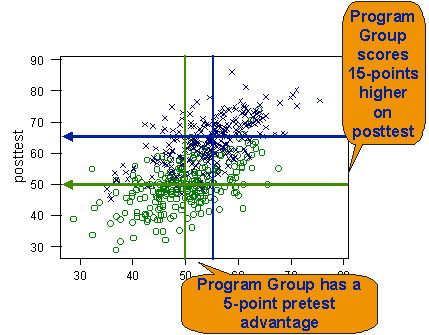## A Simulated Example

To see what happens when we use the ANCOVA analysis on data from a NEGD, I created a computer simulation to generate hypothetical data. I created 500 hypothetical persons, with 250 in the program and 250 in the comparison condition. Because this is a nonequivalent design, I made the groups nonequivalent on the pretest by adding five points to each program group person’s pretest score. Then, I added 15 points to each program person’s posttest score. When we take the initial 5-point advantage into account, we should find a 10 point program effect. The bivariate plot shows the data from this simulation.

I then analyzed the data with the ANCOVA model. Remember that the way I set this up I should observe approximately a 10-point program effect if the ANCOVA analysis works correctly. The results are presented in the table.

In this analysis, I put in three scores for each person: a pretest score (X), a posttest score (Y) and either a 0 or 1 to indicate whether the person was in the program (Z=1) or comparison (Z=0) group. The table shows the equation that the ANCOVA model estimates. The equation has the three values I put in, (X, Y and Z) and the three coefficients that the program estimates. The key coefficient is the one next to the program variable Z. This coefficient estimates the average difference between the program and comparison groups (because it’s the coefficient paired with the dummy variable indicating what group the person is in). The value should be 10 because I put in a 10 point difference. In this analysis, the actual value I got was 11.3 (or 11.2818, to be more precise). Well, that’s not too bad, you might say. It’s fairly close to the 10-point effect I put in. But we need to determine if the obtained value of 11.2818 is statistically different from the true value of 10. To see whether it is, we have to construct a confidence interval around our estimate and examine the difference between 11.2818 and 10 relative to the variability in the data. Fortunately the program does this automatically for us. If you look in the table, you’ll see that the third line shows the coefficient associated with the difference between the groups, the standard error for that coefficient (an indicator of variability), the t-value, and the probability value. All the t-value shows is that the coefficient of 11.2818 is statistically different from zero. But we want to know whether it is different from the true treatment effect value of 10. To determine this, we can construct a confidence interval around the t-value, using the standard error. We know that the 95% confidence interval is the coefficient plus or minus two times the standard error value. The calculation shows that the 95% confidence interval for our 11.2818 coefficient is 10.1454 to 12.4182. Any value falling within this range can’t be considered different beyond a 95% level from our obtained value of 11.2818. But the true value of 10 points falls outside the range. In other words, our estimate of 11.2818 is significantly different from the true value. In still other words, the results of this analysis are biased – we got the wrong answer. In this example, our estimate of the program effect is significantly larger than the true program effect (even though the difference between 10 and 11.2818 doesn’t seem that much larger, it exceeds chance levels). So, we have a problem when we apply the analysis model that our intuition tells us makes the most sense for the NEGD. To understand why this bias occurs, we have to look a little more deeply at how the statistical analysis works in relation to the NEGD.

$$y_{i}=18.7+.626 X_{i}+11.3 Z_{i}$$

$$\begin{array} {lrrrr} \text{Predictor}&\text{Coef}&\text {StErr}&\text{t}&\text{p}\\ \hline \text { Constant } & 18.714 & 1.969 & 9.50 & 0.000 \\ \text { pretest } & 0.62600 & 0.03864 & 16.20 & 0.000 \\ \text { Group } & 11.2818 & 0.5682 & 19.85 & 0.000 \end{array}$$

\begin{aligned} \mathrm{Cl}_{.95\left(\beta_{2}=10\right)} &=\beta_{2} \pm 2 \mathrm{SE}\left(\beta_{2}\right) \\ =& 11.2818 \pm 2(.5682) \\ =& 11.2818 \pm 1.1364 \end{aligned}

$$C I=10.1454 \text { to } 12.4182$$

## The Problem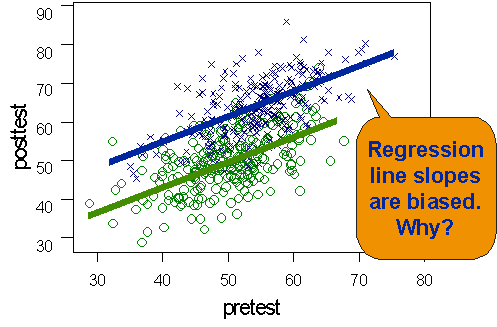Why is the ANCOVA analysis biased when used with the NEGD? And, why isn’t it biased when used with a pretest-posttest randomized experiment? Actually, there are several things happening to produce the bias, which is why it’s somewhat difficult to understand (and counterintuitive). Here are the two reasons we get a bias:

• pretest measurement error which leads to the attenuation or “flattening” of the slopes in the regression lines
• group nonequivalence

The first problem actually also occurs in randomized studies, but it doesn’t lead to biased treatment effects because the groups are equivalent (at least probabilistically). It is the combination of both these conditions that causes the problem. And, understanding the problem is what leads us to a solution in this case.

### Regression and Measurement Error

We begin our attempt to understand the source of the bias by considering how error in measurement affects regression analysis. We’ll consider three different measurement error scenarios to see what error does. In all three scenarios, we assume that there is no true treatment effect, that the null hypothesis is true. The first scenario is the case of no measurement error at all. In this hypothetical case, all of the points fall right on the regression lines themselves. The second scenario introduces measurement error on the posttest, but not on the pretest. The figure shows that when we have posttest error, we are disbursing the points vertically – up and down – from the regression lines. Imagine a specific case, one person in our study. With no measurement error the person would be expected to score on the regression line itself. With posttest measurement error, they would do better or worse on the posttest than they should. And, this would lead their score to be displaced vertically. In the third scenario we have measurement error only on the pretest. It stands to reason that in this case we would be displacing cases horizontally – left and right – off of the regression lines. For these three hypothetical cases, none of which would occur in reality, we can see how data points would be disbursed.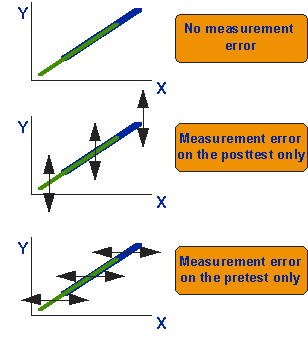### How Regression Fits Lines

Regression analysis is a least squares analytic procedure. The actual criterion for fitting the line is to fit it so that you minimize the sum of the squares of the residuals from the regression line. Let’s deconstruct this sentence a bit. The key term is “residual.” The residual is the vertical distance from the regression line to each point.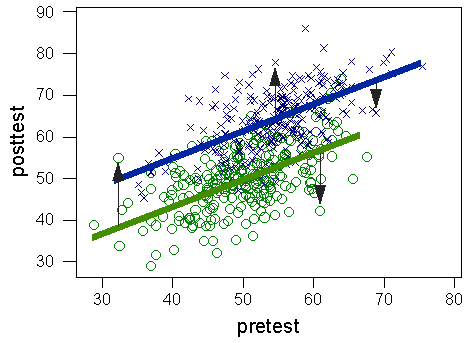The graph shows four residuals, two for each group. Two of the residuals fall above their regression line and two fall below. What is the criterion for fitting a line through the cloud of data points? Take all of the residuals within a group (we’ll fit separate lines for the program and comparison group). If they are above the line they will be positive and if they’re below they’ll be negative values. Square all the residuals in the group. Compute the sum of the squares of the residuals – just add them. That’s it. Regression analysis fits a line through the data that yields the smallest sum of the squared residuals. How it does this is another matter. But you should now understand what it’s doing. The key thing to notice is that the regression line is fit in terms of the residuals and the residuals are vertical displacements from the regression line.

### How Measurement Error Affects Slope

Now we’re ready to put the ideas of the previous two sections together. Again, we’ll consider our three measurement error scenarios described above. When there is no measurement error, the slopes of the regression lines are unaffected. The figure shown earlier shows the regression lines in this no error condition. Notice that there is no treatment effect in any of the three graphs shown in the figure (there would be a treatment effect only if there was a vertical displacement between the two lines). Now, consider the case where there is measurement error on the posttest. Will the slopes be affected? The answer is no. Why? Because in regression analysis we fit the line relative to the vertical displacements of the points. Posttest measurement error affects the vertical dimension, and, if the errors are random, we would get as many residuals pushing up as down and the slope of the line would, on average, remain the same as in the null case. There would, in this posttest measurement error case, be more variability of data around the regression line, but the line would be located in the same place as in the no error case.

Now, let’s consider the case of measurement error on the pretest. In this scenario, errors are added along the horizontal dimension. But regression analysis fits the lines relative to vertical displacements. So how will this affect the slope? The figure illustrates what happens. If there was no error, the lines would overlap as indicated for the null case in the figure. When we add in pretest measurement error, we are in effect elongating the horizontal dimension without changing the vertical. Since regression analysis fits to the vertical, this would force the regression line to stretch to fit the horizontally elongated distribution. The only way it can do this is by rotating around its center point. The result is that the line has been “flattened” or “attenuated” – the slope of the line will be lower when there is pretest measurement error than it should actually be. You should be able to see that if we flatten the line in each group by rotating it around its own center that this introduces a displacement between the two lines that was not there originally. Although there was no treatment effect in the original case, we have introduced a false or “pseudo” effect. The biased estimate of the slope that results from pretest measurement error introduces a phony treatment effect. In this example, it introduced an effect where there was none. In the simulated example shown earlier, it exaggerated the actual effect that we had constructed for the simulation.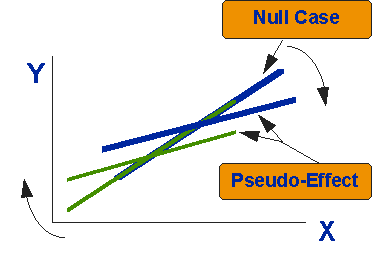### Why Doesn’t the Problem Occur in Randomized Designs?

So, why doesn’t this pseudo-effect occur in the randomized Analysis of Covariance design? The next figure shows that even in the randomized design, pretest measurement error does cause the slopes of the lines to be flattened. But, we don’t get a pseudo-effect in the randomized case even though the attenuation occurs. Why? Because in the randomized case the two groups are equivalent on the pretest – there is no horizontal difference between the lines. The lines for the two groups overlap perfectly in the null case. So, when the attenuation occurs, it occurs the same way in both lines and there is no vertical displacement introduced between the lines. Compare this figure to the one above. You should now see that the difference is that in the NEGD case above we have the attenuation of slopes and the initial nonequivalence between the groups. Under these circumstances the flattening of the lines introduces a displacement. In the randomized case we also get the flattening, but there is no displacement because there is no nonequivalence between the groups initially.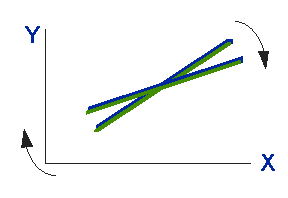### Summary of the Problem

So where does this leave us? The ANCOVA statistical model seemed at first glance to have all of the right components to correctly model data from the NEGD. But we found that it didn’t work correctly – the estimate of the treatment effect was biased. When we examined why, we saw that the bias was due to two major factors: the attenuation of slope that results from pretest measurement error coupled with the initial nonequivalence between the groups. The problem is not caused by posttest measurement error because of the criterion that is used in regression analysis to fit the line. It does not occur in randomized experiments because there is no pretest nonequivalence. We might also guess from these arguments that the bias will be greater with greater nonequivalence between groups – the less similar the groups the bigger the problem. In real-life research, as opposed to simulations, you can count on measurement error on all measurements – we never measure perfectly. So, in nonequivalent groups designs we now see that the ANCOVA analysis that seemed intuitively sensible can be expected to yield incorrect results!

## The Solution

Now that we understand the problem in the analysis of the NEGD, we can go about trying to fix it. Since the problem is caused in part by measurement error on the pretest, one way to deal with it would be to address the measurement error issue. If we could remove the pretest measurement error and approximate the no pretest error case, there would be no attenuation or flattening of the regression lines and no pseudo-effect introduced. To see how we might adjust for pretest measurement error, we need to recall what we know about measurement error and its relation to reliability of measurement.

Recall from reliability theory and the idea of true score theory that reliability can be defined as the ratio:

\begin{aligned} \frac{\ { var(T) }}{var(T) + var(e)} \\\end{aligned}

where T is the true ability or level on the measure and e is measurement error. It follows that the reliability of the pretest is directly related to the amount of measurement error. If there is no measurement error on the pretest, the var(e) term in the denominator is zero and reliability = 1. If the pretest is nothing but measurement error, the var(T) term is zero and the reliability is 0. That is, if the measure is nothing but measurement error, it is totally unreliable. If half of the measure is true score and half is measurement error, the reliability is .5. This shows that there is a direct relationship between measurement error and reliability – reliability reflects the proportion of measurement error in your measure. Since measurement error on the pretest is a necessary condition for bias in the NEGD (if there is no pretest measurement error there is no bias even in the NEGD), if we correct for the measurement error we correct for the bias. But, we can’t see measurement error directly in our data (remember, only God can see how much of a score is True Score and how much is error). However, we can estimate the reliability. Since reliability is directly related to measurement error, we can use the reliability estimate as a proxy for how much measurement error is present. And, we can adjust pretest scores using the reliability estimate to correct for the attenuation of slopes and remove the bias in the NEGD.

### The Reliability-Corrected ANCOVA

We’re going to solve the bias in ANCOVA treatment effect estimates for the NEGD using a “reliability” correction that will adjust the pretest for measurement error. The figure shows what a reliability correction looks like. The top graph shows the pretest distribution as we observe it, with measurement error included in it. Remember that I said above that adding measurement error widens or elongates the horizontal dimension in the bivariate distribution. In the frequency distribution shown in the top graph, we know that the distribution is wider than it would be if there was no error in measurement. The second graph shows that what we really want to do in adjusting the pretest scores is to squeeze the pretest distribution inwards by an amount proportionate to the amount that measurement error elongated widened it. We will do this adjustment separately for the program and comparisons groups. The third graph shows what effect “squeezing” the pretest would have on the regression lines – It would increase their slopes rotating them back to where they truly belong and removing the bias that was introduced by the measurement error. In effect, we are doing the opposite of what measurement error did so that we can correct for the measurement error.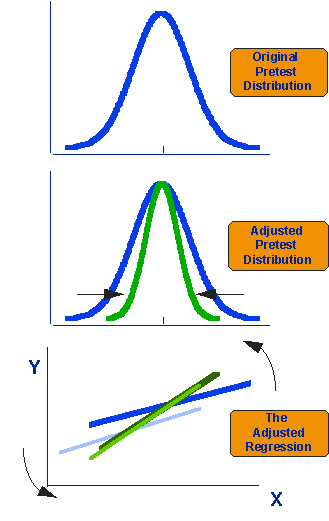All we need to know is how much to squeeze the pretest distribution in to correctly adjust for measurement error. The answer is in the reliability coefficient. Since reliability is an estimate of the proportion of your measure that is true score relative to error, it should tell us how much we have to “squeeze.” In fact, the formula for the adjustment is very simple:

$$X_{\mathrm{adj}}=\bar{X}+r(X-\bar{X})$$

Where:

• Xadj = adjusted pretest value,

• X̄ = original pretest value,

• r = reliability

The idea in this formula is that we are going to construct new pretest scores for each person. These new scores will be “adjusted” for pretest unreliability by an amount proportional to the reliability. Each person’s score will be closer to the pretest mean for that group. The formula tells us how much closer. Let’s look at a few examples. First, let’s look at the case where there is no pretest measurement error. Here, reliability would be 1. In this case, we actually don’t want to adjust the data at all. Imagine that we have a person with a pretest score of 40, where the mean of the pretest for the group is 50. We would get an adjusted score of:

\begin{aligned} \mathrm{X}_{adj} &=50 + 1(40-50) \\ \mathrm{X}_{adj} &=50 + 1(-10) \\ \mathrm{X}_{adj} &=50 - 10 \\ \mathrm{X}_{adj} &=40 \end{aligned}

Or, in other words, we wouldn’t make any adjustment at all. That’s what we want in the no measurement error case.

Now, let’s assume that reliability was relatively low, say .5. For a person with a pretest score of 40 where the group mean is 50, we would get:

\begin{aligned} \mathrm{X}_{adj} &=50 + .5(40-50) \\ \mathrm{X}_{adj} &=50 + .5(-10) \\ \mathrm{X}_{adj} &=50 - 5 \\ \mathrm{X}_{adj} &=45 \end{aligned}

Or, when reliability is .5, we would move the pretest score halfway in towards the mean (halfway from its original value of 40 towards the mean of 50, or to 45).

Finally, let’s assume that for the same case the reliability was stronger at .8. The reliability adjustment would be:

\begin{aligned} \mathrm{X}_{adj} &=50 + .8(40-50) \\ \mathrm{X}_{adj} &=50 + .8(-10) \\ \mathrm{X}_{adj} &=50 - 8 \\ \mathrm{X}_{adj} &=42 \end{aligned}

That is, with reliability of .8 we would want to move the score in 20% towards its mean (because if reliability is .8, the amount of the score due to error is 1 -.8 = .2).

You should be able to see that if we make this adjustment to all of the pretest scores in a group, we would be “squeezing” the pretest distribution in by an amount proportionate to the measurement error (1 - reliability). It’s important to note that we need to make this correction separately for our program and comparison groups.

We’re now ready to take this adjusted pretest score and substitute it for the original pretest score in our ANCOVA model:

$$y_{i}=\beta_{0}+\beta_{1} X_{adj}+\beta_{2} Z_{i}+e_{i}$$

Where:

• yi = outcome score for the ith unit,

• β0 = coefficient for the intercept,

• β1 = pretest coefficient,

• β2 = mean difference for treatment,

• Xadj = covariate adjusted for unreliability,

• Zi = dummy variable for treatment (0 = control, 1 = treatment),

• ei = residual for the ith unit

Notice that the only difference is that we’ve changed the X in the original ANCOVA to the term Xadj.

## The Simulation Revisited

So, let’s go see how well our adjustment works. We’ll use the same simulated data that we used earlier. The results are:

$$y_{i}=-3.14+1.06 X_{a d j}+9.30 Z_{i}$$

$$\begin{array}{lrrcl} \text { Predictor } & \text { Coef } & \text { StErr } & \text { t } & \text { p } \\ \hline \text { Constant } & -3.141 & 3.300 & -0.95 & 0.342 \\ \text { adjpre } & 1 . 0 6 3 1 6 & 0 . 0 6 5 5 7 & 1 6 . 2 1 & 0 . 0 0 0 \\ \text { Group } & 9 . 3 0 4 8 & 0 . 6 1 6 6 & 1 5 . 0 9 & 0 . 0 0 0 \end{array}$$

\begin{aligned} \mathrm{Cl}_{.95\left(\beta_{2}=10\right)} &=\beta_{2} \pm 2 \mathrm{SE}\left(\beta_{2}\right) \\ =& 9.3048 \pm 2(.6166) \\ =& 9.3048 \pm 1.2332 \end{aligned}

$$C I=8.0716 \text { to } 10.5380$$

This time we get an estimate of the treatment effect of 9.3048 (instead of 11.2818). This estimate is closer to the true value of 10 points that we put into the simulated data. And, when we construct a 95% confidence interval for our adjusted estimate, we see that the true value of 10 falls within the interval. That is, the analysis estimated a treatment effect that is not statistically different from the true effect – it is an unbiased estimate.

You should also compare the slope of the lines in this adjusted model with the original slope. Now, the slope is nearly 1 at 1.06316, whereas before it was .626 – considerably lower or “flatter.” The slope in our adjusted model approximates the expected true slope of the line (which is 1). The original slope showed the attenuation that the pretest measurement error caused.

So, the reliability-corrected ANCOVA model is used in the statistical analysis of the NEGD to correct for the bias that would occur as a result of measurement error on the pretest.

## Which Reliability To Use?

There’s really only one more major issue to settle in order to finish the story. We know from reliability theory that we can’t calculate the true reliability, we can only estimate it. There a variety of reliability estimates and they’re likely to give you different values. Cronbach’s Alpha tends to be a high estimate of reliability. The test-retest reliability tends to be a lower-bound estimate of reliability. So which do we use in our correction formula? The answer is: both! When analyzing data from the NEGD it’s safest to do two analyses, one with an upper-bound estimate of reliability and one with a lower-bound one. If we find a significant treatment effect estimate with both, we can be fairly confident that we would have found a significant effect in data that had no pretest measurement error.

This certainly doesn’t feel like a very satisfying conclusion to our rather convoluted story about the analysis of the NEGD, and it’s not. In some ways, I look at this as the price we pay when we give up random assignment and use intact groups in a NEGD – our analysis becomes more complicated as we deal with adjustments that are needed, in part, because of the nonequivalence between the groups. Nevertheless, there are also benefits in using nonequivalent groups instead of randomly assigning. You have to decide whether the tradeoff is worth it.